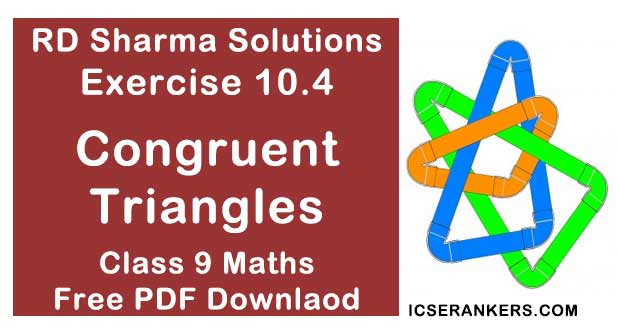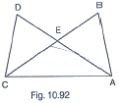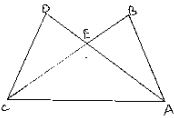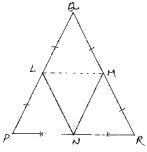# Chapter 10 Congruent Triangles RD Sharma Solutions Exercise 10.4 Class 9 MathsChapter Name RD Sharma Chapter 10 Congruent Triangles Exercise 10.4 Book Name RD Sharma Mathematics for Class 10 Other Exercises Exercise 10.1Exercise 10.2Exercise 10.3Exercise 10.5Exercise 10.6 Related Study NCERT Solutions for Class 10 Maths

### Exercise 10.4 Solutions

1. In Fig. 10.92, it is given that AB = CD and AD = BC. Prove that ΔADC ≅ ΔCBA.SolutionGiven that in the figure AB = CD and AD = BC.
We have to prove
Now,
We have
AB = CD  [Given]
And AC = AC  [Common side]
So, by SSS congruence criterion, we have
∴ Hence proved

2. In a ΔPQR, if PQ = QR and L, M and N are the mid - points of the sides PQ, QR and RP respectively. Prove that LN  = MN.
SolutionGiven that in ΔPQR, PQ = QR and L, M and N are mid - points of PQ, QR and RP respectively
We have to prove LN = MN.
Join L and M, M and N, N and L
We have
PL = LQ, QM = MR and RN  = NP
[∵ L, M and N are mid - points of PQ, QR and RP respectively]
And also,
PQ = QR ⇒ PL = LQ = QM = MR = PQ/2 = QR/2 ...(1)
Using mid - point theorem, we have
MN || PQ and MN = (1/2)(PQ)
⇒ [MN = PL = LQ]  ...(2)
Similarly, we have
LN || QR and LN = (1/2)(QR)
⇒ LN = QM = MR
From equation (1), (2) and (3), we have
PL = LQ = QM  = MR = MN = LN
∴ LN = MN